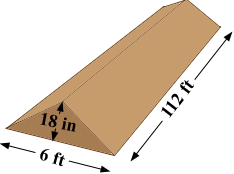SEARCH HOMEMath Central Quandaries & QueriesQuestion from jim, a parent: a berm 6 ft wide x 112 ft long 18 inches high at center x 18 inches down to 0 front and back as a taper...how much soil do I need?Hi Jim,

Is this what your berm looks like?If so then the volume is the area of the triangular cross-section, times the length. Since I expect you want the volume in cubic yards I would start by converting all the dimensions to yards.

The triangular cross-section then has a base of length 6/3 = 2 yards and a height of 18.36 = 1/2 yards. The area of this triangle is then

1/2 × base × height = 1/2 × 2 × 1/2 = 1/2 square yards.

The length of the berm is 112/3 yards so the volume is

(area of the triangle) × length = 1/2 × 112/3 = 18 2/3 cubic yards.

PennyMath Central is supported by the University of Regina and The Pacific Institute for the Mathematical Sciences.